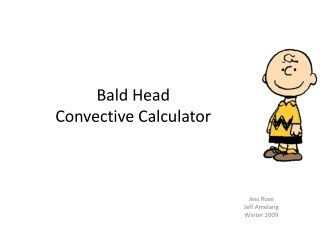Download Presentation# Bald Head Convective Calculator - PowerPoint PPT PresentationDownload Presentation- - - - - - - - - - - - - - - - - - - - - - - - - - - E N D - - - - - - - - - - - - - - - - - - - - - - - - - - -
##### Presentation Transcript

1. Bald Head Convective Calculator Jess Rose Jeff Amelang Winter 2009

2. Calculator Functionality Goal: Create a calculator that estimates skin temperature of a bald head under three different convective conditions: • Free convection • Forced convection (windy) • Forced convection with a beanie

3. Basic Assumptions • Head is modeled as a sphere of constant temperature with a layer of tissue • Steady state • One Dimensional Radial Conduction • Constant Properties • Neglect Radiation • Properties calculated using the source code of the thermal properties calculator tissue T_c = 98°F T = 0.25 in T_inf D = 7 in

4. Free Convection Convective coefficient is calculated using Free convection on a sphere Total thermal circuit Partial thermal circuit

5. Forced Convection (windy) Convective coefficient is calculated using Forced convection on a sphere Total thermal circuit Partial thermal circuit Includes Free Convection if .1 < abs(Gr/Re^2 ) < 10

6. Forced Convection with Beanie Convective coefficient is calculated using Forced convection on a sphere Y Total thermal circuit Partial thermal circuit Assumptions: • Two parallel thermal circuits • Infinite Contact Resistance between hemispheres

7. The Calculator

8. Improvements • Approximate head with volumetric head generation instead of a constant temperature • Eliminate assumption of infinite contact resistance between hemispheres with beanie • Include radiation

9. Conclusions • When there is convective heating of Charlie Brown’s head, the beanie keeps the skin surface temperature lower, oddly enough. • Changing the conductive coefficient of the tissue has a significant effect on the temperature of the skin.